Next: Estimation Up: Basic Concepts Previous: Image sampling

Noise distributions

Besides gross errors which are discussed in Section 2.2.1 the two main sources of noise in a frame come from the detector system N and from photon shot-noise of the image intensity I (see Equation 2.1). It is assumed that the digitalization is done with sufficiently high resolution to resolve the noise. If not, the quantization of output values gives raise to additional noise and errors.

A large number of independent noise sources from different electronics components normally contributes to the system noise of a detector. Using the central limit theorem, the total noise can be approximated by a Gaussian or normal distribution which has the frequency function :(2.3)

where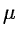and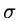are mean and standard deviation, respectively. The photon noise of a source is Poisson distributed with the probability density PP for a given number of photons n :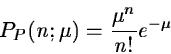(2.4)

where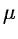is the mean intensity of the source. It can be approximated with a Gaussian distribution whenbecomes large. For photon counting devices the number of events is normally so small that Equation 2.4 must be used while Gaussian approximation often can be used for integrating systems (e.g. CCD's).

In the statistical analysis of the probability distribution of data several estimators based on moments are used. The rth moment mr about the mean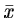and its dimensional form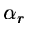are defined as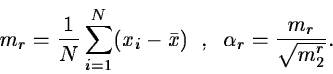(2.5)

The second moment is the variance while first is always zero. The general shape of a distribution is characterized by the skewness which denotes its asymmetry (i.e. its third moment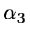) and the kurtosis showing how peaked it is (i.e. its fourth moment). For a normal distribution, these moments are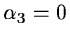and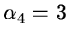while for a Poisson distribution are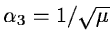and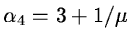. Besides these moments other estimators are used to describe a distribution e.g. median and mode. The median of a distribution is defined as the value which has equally many values above and below it while a mode is the local maximum of the probability density function.Next: Estimation Up: Basic Concepts Previous: Image sampling
Petra Nass
1999-06-15